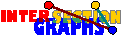# The Helly property

It turns out that the restriction to intersection graphs of Helly hypergraphs is the most important reason for making things (first of all, recognition) easier. We may not know all star graphs, but many of them.

Now, many natural properties P have the property that every system of P-sets has the Helly-property. Examples are intervals of the real line, or more generally, d-dimensional boxes Also, subtrees of a given tree fulfill the Helly-property. This property is named after the Austrian mathematician Eduard Helly. Helly showed in 1923 that whenever every d+1 sets of a collection of convex sets in Rd have nonempty intersection, then the total intersection of these sets must be nonempty too. That coincides with our definition only for d=2, but see here for applications of this so-called k-Helly property.

The dual notion of `Helly' is `conformal'. A hypergraph H is called conformal if its dual H* has the Helly-property. Another formulation is: Every clique of the underlying graph of H should be covered by some hyperedge, that is, The clique hypergraph of the underlying graph of H should be contained in H. Now it is very easy to convince you that every graph is not only an intersection graph, but even an intersection graph of some Helly-hypergraph, since every graph is the underlying graph of its own (conformal) clique hypergraph.

See examples how to recognize interval graphs respectively line graphs with this approach. However, the approach fails for intersection graphs of boxes in R2.

In models with Helly property, the topology of the union of all sets considered is related to the topology of the intersection graph. Or go back to the start page for intersection graphs.

Erich Prisner